# 8.3 The parabola  (Page 7/11)

 Page 7 / 11

${\left(y+4\right)}^{2}=16\left(x+4\right)$

${y}^{2}+12x-6y+21=0$

${\left(y-3\right)}^{2}=-12\left(x+1\right),V:\left(-1,3\right);F:\left(-4,3\right);d:x=2$

${x}^{2}-4x-24y+28=0$

$5{x}^{2}-50x-4y+113=0$

${\left(x-5\right)}^{2}=\frac{4}{5}\left(y+3\right),V:\left(5,-3\right);F:\left(5,-\frac{14}{5}\right);d:y=-\frac{16}{5}$

${y}^{2}-24x+4y-68=0$

${x}^{2}-4x+2y-6=0$

${\left(x-2\right)}^{2}=-2\left(y-5\right),V:\left(2,5\right);F:\left(2,\frac{9}{2}\right);d:y=\frac{11}{2}$

${y}^{2}-6y+12x-3=0$

$3{y}^{2}-4x-6y+23=0$

${\left(y-1\right)}^{2}=\frac{4}{3}\left(x-5\right),V:\left(5,1\right);F:\left(\frac{16}{3},1\right);d:x=\frac{14}{3}$

${x}^{2}+4x+8y-4=0$

## Graphical

For the following exercises, graph the parabola, labeling the focus and the directrix.

$x=\frac{1}{8}{y}^{2}$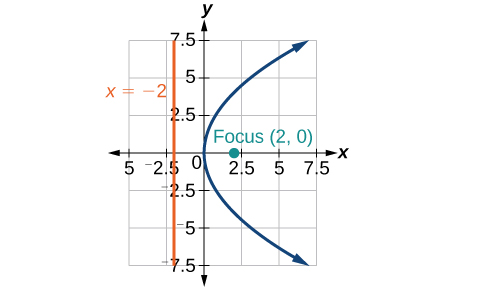$y=36{x}^{2}$

$y=\frac{1}{36}{x}^{2}$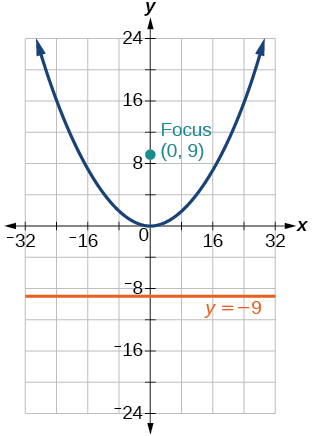$y=-9{x}^{2}$

${\left(y-2\right)}^{2}=-\frac{4}{3}\left(x+2\right)$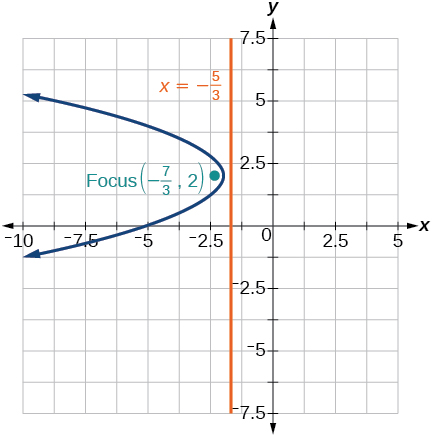$-5{\left(x+5\right)}^{2}=4\left(y+5\right)$

$-6{\left(y+5\right)}^{2}=4\left(x-4\right)$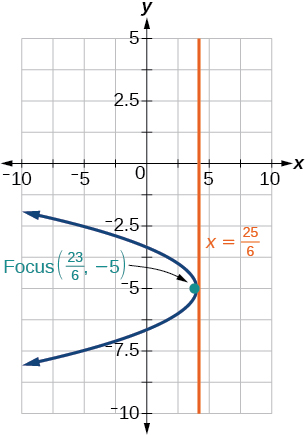${y}^{2}-6y-8x+1=0$

${x}^{2}+8x+4y+20=0$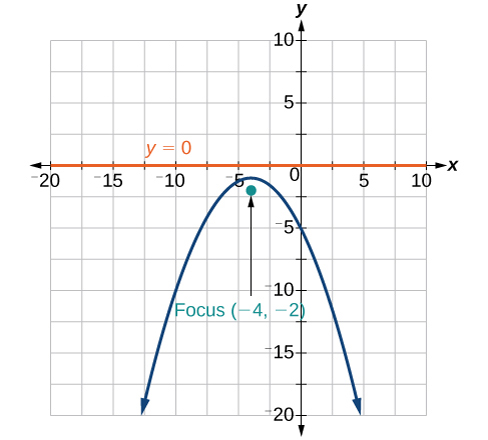$3{x}^{2}+30x-4y+95=0$

${y}^{2}-8x+10y+9=0$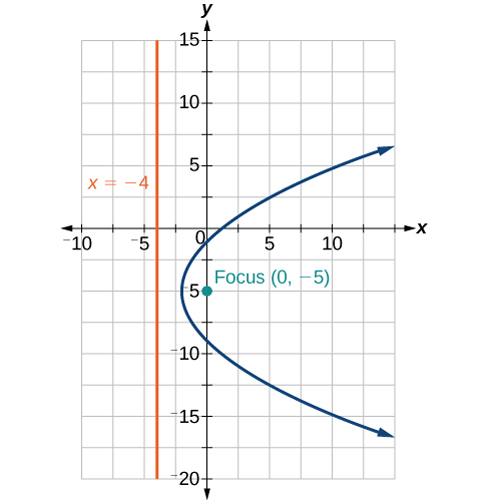${x}^{2}+4x+2y+2=0$

${y}^{2}+2y-12x+61=0$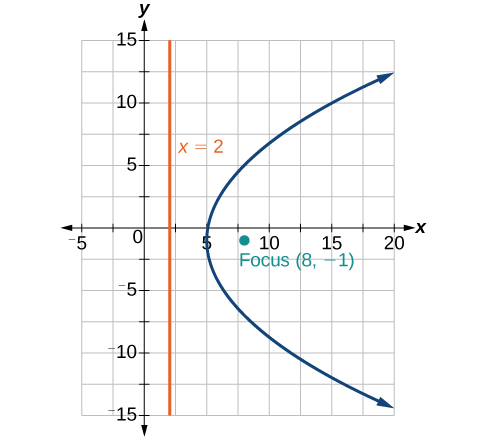$-2{x}^{2}+8x-4y-24=0$

For the following exercises, find the equation of the parabola given information about its graph.

Vertex is $\text{\hspace{0.17em}}\left(0,0\right);$ directrix is $\text{\hspace{0.17em}}y=4,$ focus is $\text{\hspace{0.17em}}\left(0,-4\right).$

${x}^{2}=-16y$

Vertex is $\text{\hspace{0.17em}}\left(0,0\right);\text{\hspace{0.17em}}$ directrix is $\text{\hspace{0.17em}}x=4,$ focus is $\text{\hspace{0.17em}}\left(-4,0\right).$

Vertex is $\text{\hspace{0.17em}}\left(2,2\right);\text{\hspace{0.17em}}$ directrix is $\text{\hspace{0.17em}}x=2-\sqrt{2},$ focus is $\text{\hspace{0.17em}}\left(2+\sqrt{2},2\right).$

${\left(y-2\right)}^{2}=4\sqrt{2}\left(x-2\right)$

Vertex is $\text{\hspace{0.17em}}\left(-2,3\right);\text{\hspace{0.17em}}$ directrix is $\text{\hspace{0.17em}}x=-\frac{7}{2},$ focus is $\text{\hspace{0.17em}}\left(-\frac{1}{2},3\right).$

Vertex is $\text{\hspace{0.17em}}\left(\sqrt{2},-\sqrt{3}\right);$ directrix is $\text{\hspace{0.17em}}x=2\sqrt{2},$ focus is $\text{\hspace{0.17em}}\left(0,-\sqrt{3}\right).$

${\left(y+\sqrt{3}\right)}^{2}=-4\sqrt{2}\left(x-\sqrt{2}\right)$

Vertex is $\text{\hspace{0.17em}}\left(1,2\right);\text{\hspace{0.17em}}$ directrix is $\text{\hspace{0.17em}}y=\frac{11}{3},$ focus is $\text{\hspace{0.17em}}\left(1,\frac{1}{3}\right).$

For the following exercises, determine the equation for the parabola from its graph.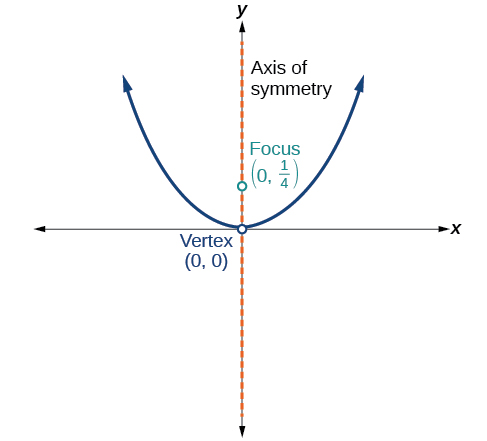${x}^{2}=y$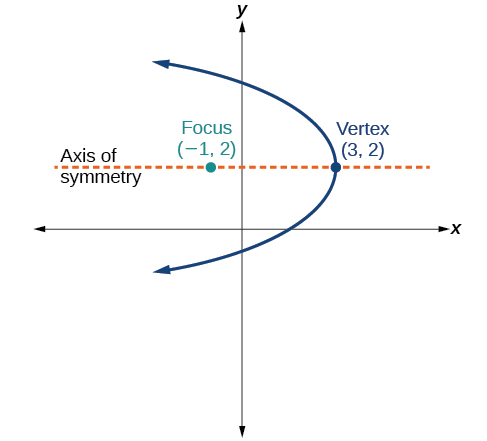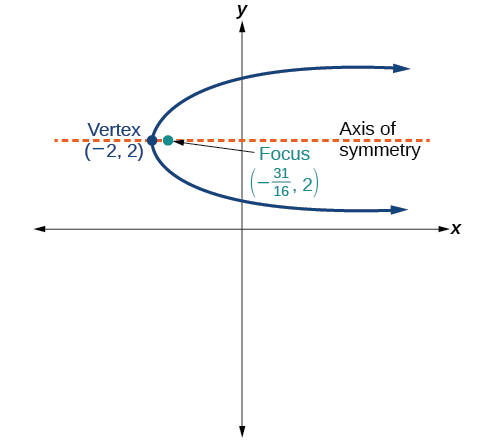${\left(y-2\right)}^{2}=\frac{1}{4}\left(x+2\right)$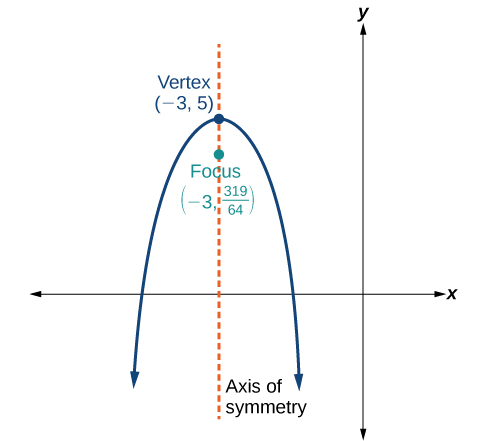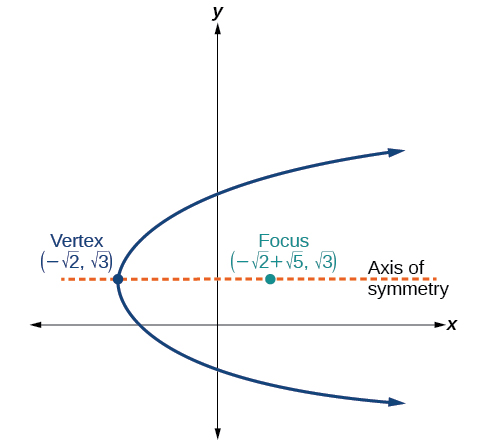${\left(y-\sqrt{3}\right)}^{2}=4\sqrt{5}\left(x+\sqrt{2}\right)$

## Extensions

For the following exercises, the vertex and endpoints of the latus rectum of a parabola are given. Find the equation.

${y}^{2}=-8x$

${\left(y+1\right)}^{2}=12\left(x+3\right)$

## Real-world applications

The mirror in an automobile headlight has a parabolic cross-section with the light bulb at the focus. On a schematic, the equation of the parabola is given as $\text{\hspace{0.17em}}{x}^{2}=4y.\text{\hspace{0.17em}}$ At what coordinates should you place the light bulb?

$\left(0,1\right)$

If we want to construct the mirror from the previous exercise such that the focus is located at $\text{\hspace{0.17em}}\left(0,0.25\right),$ what should the equation of the parabola be?

A satellite dish is shaped like a paraboloid of revolution. This means that it can be formed by rotating a parabola around its axis of symmetry. The receiver is to be located at the focus. If the dish is 12 feet across at its opening and 4 feet deep at its center, where should the receiver be placed?

At the point 2.25 feet above the vertex.

Consider the satellite dish from the previous exercise. If the dish is 8 feet across at the opening and 2 feet deep, where should we place the receiver?

A searchlight is shaped like a paraboloid of revolution. A light source is located 1 foot from the base along the axis of symmetry. If the opening of the searchlight is 3 feet across, find the depth.

0.5625 feet

If the searchlight from the previous exercise has the light source located 6 inches from the base along the axis of symmetry and the opening is 4 feet, find the depth.

An arch is in the shape of a parabola. It has a span of 100 feet and a maximum height of 20 feet. Find the equation of the parabola, and determine the height of the arch 40 feet from the center.

${x}^{2}=-125\left(y-20\right),$ height is 7.2 feet

If the arch from the previous exercise has a span of 160 feet and a maximum height of 40 feet, find the equation of the parabola, and determine the distance from the center at which the height is 20 feet.

An object is projected so as to follow a parabolic path given by $\text{\hspace{0.17em}}y=-{x}^{2}+96x,$ where $\text{\hspace{0.17em}}x\text{\hspace{0.17em}}$ is the horizontal distance traveled in feet and $\text{\hspace{0.17em}}y\text{\hspace{0.17em}}$ is the height. Determine the maximum height the object reaches.

2304 feet

For the object from the previous exercise, assume the path followed is given by $\text{\hspace{0.17em}}y=-0.5{x}^{2}+80x.\text{\hspace{0.17em}}$ Determine how far along the horizontal the object traveled to reach maximum height.

what is math number
x-2y+3z=-3 2x-y+z=7 -x+3y-z=6
Need help solving this problem (2/7)^-2
x+2y-z=7
Sidiki
what is the coefficient of -4×
-1
Shedrak
the operation * is x * y =x + y/ 1+(x × y) show if the operation is commutative if x × y is not equal to -1
An investment account was opened with an initial deposit of \$9,600 and earns 7.4% interest, compounded continuously. How much will the account be worth after 15 years?
lim x to infinity e^1-e^-1/log(1+x)
given eccentricity and a point find the equiation
12, 17, 22.... 25th term
12, 17, 22.... 25th term
Akash
College algebra is really hard?
Absolutely, for me. My problems with math started in First grade...involving a nun Sister Anastasia, bad vision, talking & getting expelled from Catholic school. When it comes to math I just can't focus and all I can hear is our family silverware banging and clanging on the pink Formica table.
Carole
I'm 13 and I understand it great
AJ
I am 1 year old but I can do it! 1+1=2 proof very hard for me though.
Atone
hi
Not really they are just easy concepts which can be understood if you have great basics. I am 14 I understood them easily.
Vedant
find the 15th term of the geometric sequince whose first is 18 and last term of 387
I know this work
salma
The given of f(x=x-2. then what is the value of this f(3) 5f(x+1)
hmm well what is the answer
Abhi
If f(x) = x-2 then, f(3) when 5f(x+1) 5((3-2)+1) 5(1+1) 5(2) 10
Augustine
how do they get the third part x = (32)5/4
make 5/4 into a mixed number, make that a decimal, and then multiply 32 by the decimal 5/4 turns out to be
AJ
how
Sheref
can someone help me with some logarithmic and exponential equations.
20/(×-6^2)
Salomon
okay, so you have 6 raised to the power of 2. what is that part of your answer
I don't understand what the A with approx sign and the boxed x mean
it think it's written 20/(X-6)^2 so it's 20 divided by X-6 squared
Salomon
I'm not sure why it wrote it the other way
Salomon
I got X =-6
Salomon
ok. so take the square root of both sides, now you have plus or minus the square root of 20= x-6
oops. ignore that.
so you not have an equal sign anywhere in the original equation?
hmm
Abhi
is it a question of log
Abhi
🤔.
Abhi
I rally confuse this number And equations too I need exactly help
salma
But this is not salma it's Faiza live in lousvile Ky I garbage this so I am going collage with JCTC that the of the collage thank you my friends
salma
Commplementary angles
hello
Sherica
im all ears I need to learn
Sherica
right! what he said ⤴⤴⤴
Tamia
hii
Uday
hi
salma
hi
Ayuba
Hello
opoku
hi
Ali
greetings from Iran
Ali
salut. from Algeria
Bach
hi
NharnharByBy